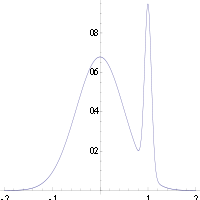# Maximum a posteriori estimation

In Bayesian statistics, a maximum a posteriori probability (MAP) estimate is an estimate of an unknown quantity, that equals the mode of the posterior distribution. The MAP can be used to obtain a point estimate of an unobserved quantity on the basis of empirical data. It is closely related to Fisher's method of maximum likelihood (ML) estimation, but employs an augmented optimization objective which incorporates a prior distribution (that quantifies the additional information available through prior knowledge of a related event) over the quantity one wants to estimate. MAP estimation can therefore be seen as a regularization of ML estimation.

## Description

Assume that we want to estimate an unobserved population parameteron the basis of observations. Letbe the sampling distribution of, so thatis the probability ofwhen the underlying population parameter is. Then the function:is known as the likelihood function and the estimate:is the maximum likelihood estimate of.

Now assume that a prior distributionoverexists. This allows us to treatas a random variable as in Bayesian statistics. We can calculate the posterior distribution ofusing Bayes' theorem:whereis density function of,is the domain of.

The method of maximum a posteriori estimation then estimatesas the mode of the posterior distribution of this random variable:The denominator of the posterior distribution (so-called marginal likelihood) does not depend onand therefore plays no role in the optimization. Observe that the MAP estimate ofcoincides with the ML estimate when the prioris uniform (that is, a constant function).

When the loss function is of the formasgoes to 0, the Bayes estimator approaches the MAP estimator, provided that the distribution ofis unimodal. But generally a MAP estimator is not a Bayes estimator unlessis discrete.

## Computation

MAP estimates can be computed in several ways:

1. Analytically, when the mode(s) of the posterior distribution can be given in closed form. This is the case when conjugate priors are used.
2. Via numerical optimization such as the conjugate gradient method or Newton's method. This usually requires first or second derivatives, which have to be evaluated analytically or numerically.
3. Via a modification of an expectation-maximization algorithm. This does not require derivatives of the posterior density.
4. Via a Monte Carlo method using simulated annealing

## Criticism

While MAP estimation is a limit of Bayes estimators (under the 0-1 loss function), it is not very representative of Bayesian methods in general. This is because MAP estimates are point estimates, whereas Bayesian methods are characterized by the use of distributions to summarize data and draw inferences: thus, Bayesian methods tend to report the posterior mean or median instead, together with credible intervals. This is both because these estimators are optimal under squared-error and linear-error loss respectively - which are more representative of typical loss functions - and because the posterior distribution may not have a simple analytic form: in this case, the distribution can be simulated using Markov chain Monte Carlo techniques, while optimization to find its mode(s) may be difficult or impossible.An example of a density of a bimodal distribution in which the highest mode is uncharacteristic of the majority of the distribution

In many types of models, such as mixture models, the posterior may be multi-modal. In such a case, the usual recommendation is that one should choose the highest mode: this is not always feasible (global optimization is a difficult problem), nor in some cases even possible (such as when identifiability issues arise). Furthermore, the highest mode may be uncharacteristic of the majority of the posterior.

Finally, unlike ML estimators, the MAP estimate is not invariant under reparameterization. Switching from one parameterization to another involves introducing a Jacobian that impacts on the location of the maximum.

As an example of the difference between Bayes estimators mentioned above (mean and median estimators) and using a MAP estimate, consider the case where there is a need to classify inputsas either positive or negative (for example, loans as risky or safe). Suppose there are just three possible hypotheses about the correct method of classification,andwith posteriors 0.4, 0.3 and 0.3 respectively. Suppose given a new instance,,classifies it as positive, whereas the other two classify it as negative. Using the MAP estimate for the correct classifier,is classified as positive, whereas the Bayes estimators would average over all hypotheses and classifyas negative.

## Example

Suppose that we are given a sequenceof IIDrandom variables and an a priori distribution ofis given by. We wish to find the MAP estimate of. Note that the normal distribution is its own conjugate prior, so we will be able to find a closed-form solution analytically.

The function to be maximized is then given bywhich is equivalent to minimizing the following function of:Thus, we see that the MAP estimator for μ is given bywhich turns out to be a linear interpolation between the prior mean and the sample mean weighted by their respective covariances.

The case ofis called a non-informative prior and leads to an ill-defined a priori probability distribution; in this case1. Murphy, Kevin P. (2012). Machine learning : a probabilistic perspective. Cambridge, MA: MIT Press. pp. 151–152. ISBN 0262018020.
• M. DeGroot, Optimal Statistical Decisions, McGraw-Hill, (1970).
• Harold W. Sorenson, (1980) "Parameter Estimation: Principles and Problems", Marcel Dekker.